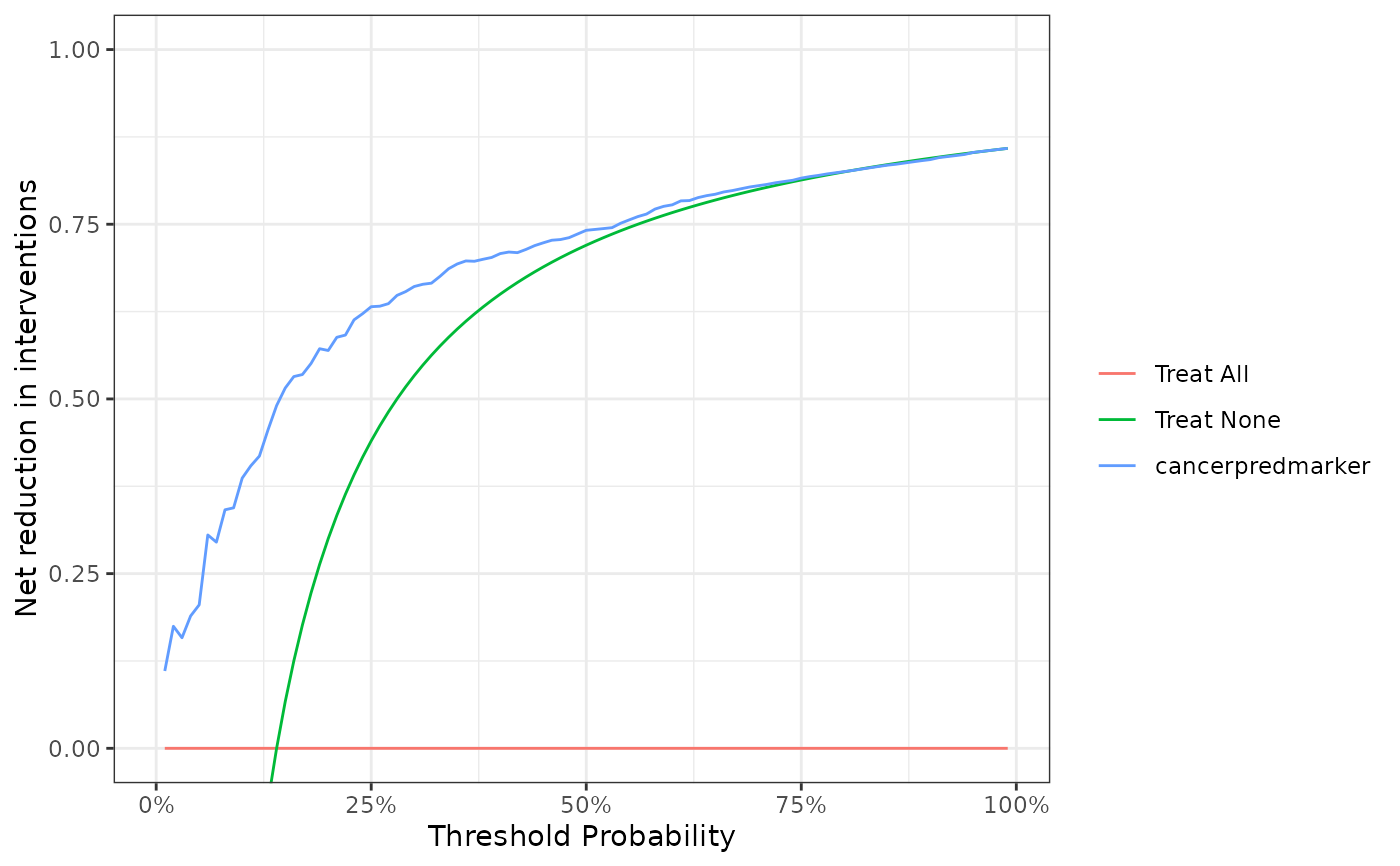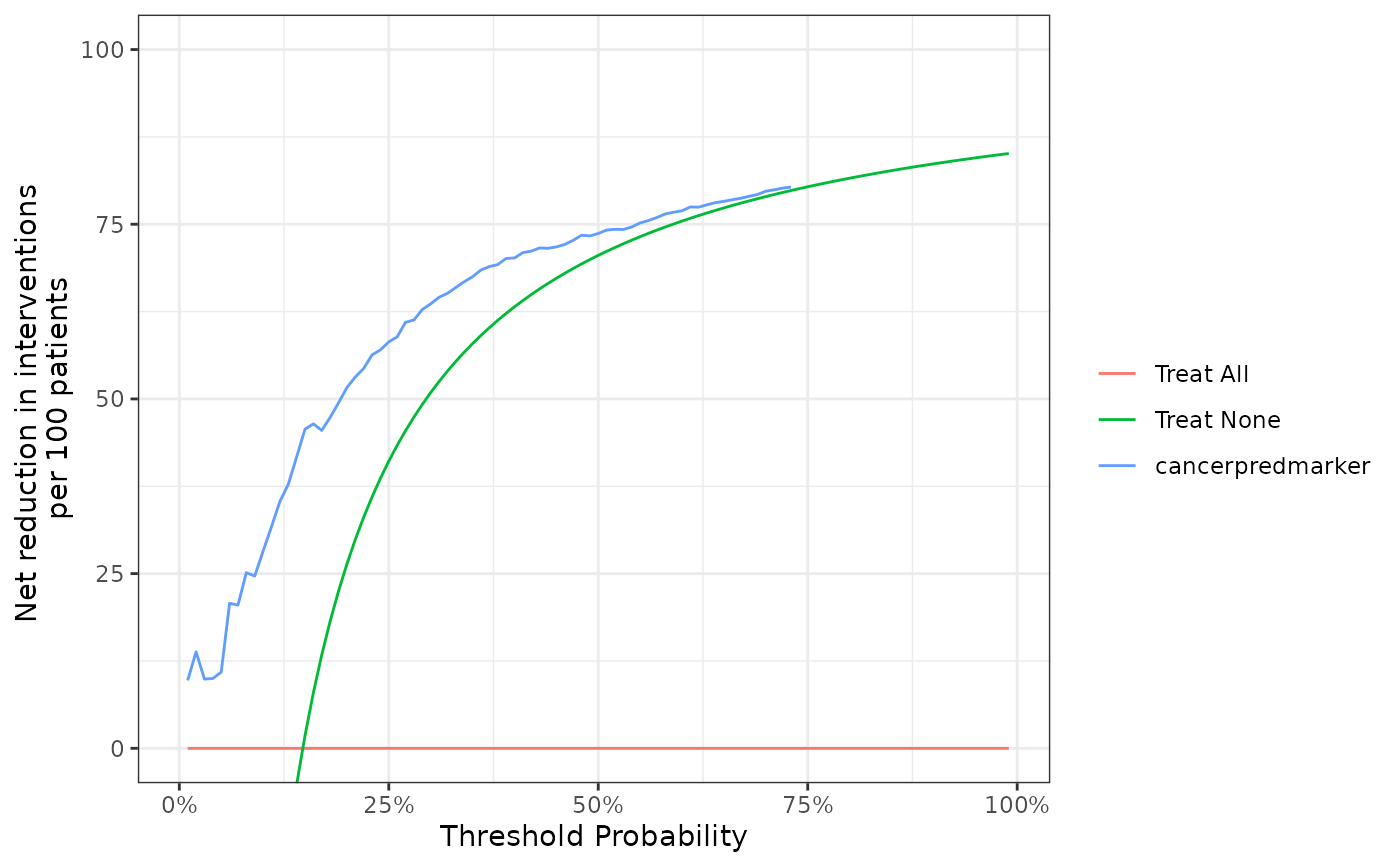Add the number of net interventions avoided to dca() object.

net_intervention_avoided(x, nper = 1)

## Arguments

x

object of class 'dca' calculated with dca()

nper

Number to report net interventions per. Default is 1

## Value

'dca' object

dca(), standardized_net_benefit(), plot.dca(), as_tibble.dca()

Daniel D Sjoberg

## Examples

dca(
cancer ~ cancerpredmarker,
data = df_binary
) %>%
net_intervention_avoided()
#> Printing with plot(x, type = 'net_intervention_avoided', smooth = FALSE, show_ggplot_code = FALSE)dca(
Surv(ttcancer, cancer) ~ cancerpredmarker,
data = df_surv,
time = 1
) %>%
net_intervention_avoided(nper = 100)
#> Printing with plot(x, type = 'net_intervention_avoided', smooth = FALSE, show_ggplot_code = FALSE)